Search

Completing the Square: Ellipse EquationsOne general format of an ellipse is ax2 + by2 + cx + dy + e = 0. But the more useful form looks quite different: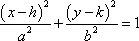...where the point (h, k) is the center of the ellipse, and the focal points and the axis lengths of the ellipse can be found from the values of a and b. But how do you convert from the general form to the useful form? By completing the square.

• Find the focus equation of the ellipse given by  4x2 + 9y2  48x + 72y + 144 = 0.

 This is your original equation.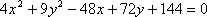Move the loose number over to the other side, and group the x-stuff and y-stuff together.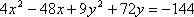Factor out whatever is on the squared terms.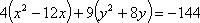Divide through by whatever you factored out of the x-stuff.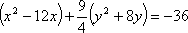Divide through by whatever you factored out of the y-stuff.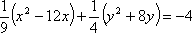Leave space in the x-stuff, the y-stuff, and on the right side. Whatever numbers are multiplied on the x-stuff and the y-stuff, put them on the spaces on the right. Take half of the coefficients of the first-degree terms (and don't forget their signs!), square them, and add the squares into the appropriate spaces on both sides of the equation.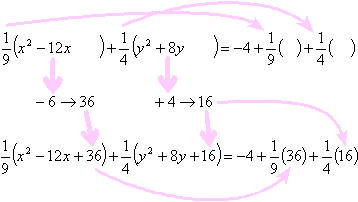Simplify on the right, and convert to squared form on the left. (This is where you use those signs you kept track of earlier.)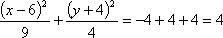Whatever value you get on the right side, divide through by it, so the right side becomes "= 1".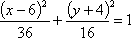The process for hyperbolas is the same, except that the signs on the x-squared and y-squared terms will be opposite; that is, while both the x-squared and y-squared terms are added in the case of ellipses (and circles), one or the other will be subtracted in the case of hyperbolas.

 Cite this article as: Stapel, Elizabeth. "Completing the Square: Ellipse Equations." Purplemath. Available from     https://www.purplemath.com/modules/sqrellps.htm. Accessed [Date] [Month] 2016

Study Skills Survey

Tutoring from Purplemath
Find a local math tutor